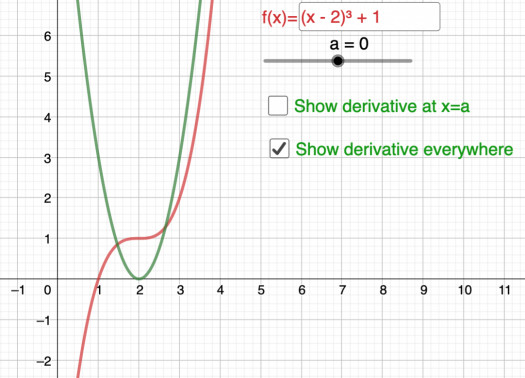# Derivative Of A Function

8 Questions | Attempts: 364Settings. How are a function and its derivative related? When does the derivative not exist?

• 1.
Check all of the options which represent the derivative of f(x) = x4.
• A.

Option 1

• B.

Option 2

• C.

Option 3

• D.

Option 4

• E.

Option 5

• F.

Option 6

• G.

Option 7

• 2.
Estimate G'(7) from the graph of G(x).
• A.

0.2

• B.

0.5

• C.

1

• D.

2

• E.

5

• 3.
At which values of x does the derivative of the graphed function not exist?
• A.

X = –6

• B.

X = –4

• C.

X = –2

• D.

X = –1

• E.

X = 0

• F.

X = 2

• G.

X = 3

• H.

X = 4

• 4.
STATEMENT: All continuous functions are differentiable.
• A.

True

• B.

False

• 5.
All differentiable functions are continuous.
• A.

True

• B.

False

• 6.
If a function has a discontinuity at x = a, then the function does not have a derivative at x = a.
• A.

True

• B.

False

• 7.
The derivative does not exist at a cusp, but it does exist at a corner.
• A.

True

• B.

False

• 8.
You have practiced applying algebraic techniques to find the derivative of functions from the definition of derivative (limit of a difference quotient).
• A.

True

• B.

False

## Related TopicsBack to top
×

Wait!
Here's an interesting quiz for you.